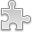# Courses / Module

Toggle Print

##NUMERICAL COMPUTATION

Module code: CS417
Credits: 5
Semester: 2
Department: COMPUTER SCIENCE
International:Overview

Topics from: Error explosion; floating point arithmetic; systems of linear and non-linear equations interpolation; numerical differentiation; ordinary differential equations; boundary value problems; Partial differential equations; non-linear equations; non standard computations. Emphasis: On applications not theory; on adapting mathematical algorithms; on error control; on programming solutions, Matlab, Java, Perl.Learning OutcomesTeaching & Learning methodsAssessmentAutumn Supplementals/ResitsTimetable